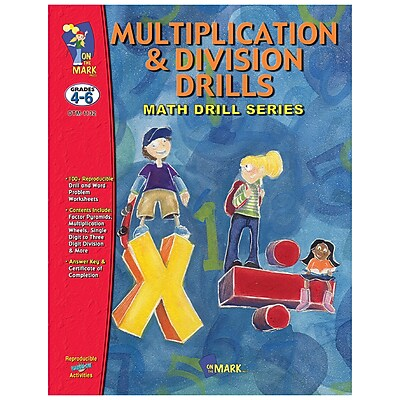# Math Drills, On The Mark Press Multiplication and Division

Item #: 846786 | Model #: OTM1132
Math Drills, On The Mark Press Multiplication and Divisionstars - based on0reviews
Get free shipping on your qualifying orders of On The Mark Press® Multiplication and Division Math Drill Book, Grades 4th - 6th.
Product ID: 846786
23.79 NewIn stock
\$23.79
Multiplication and division math drill book helps to understand pyramids and multiplication wheels and an introduction to decimals.

On The Mark Press Multiplication and division math drill book is ideal for ages 9 - 12 and grades 4th - 6th. Book helps to understand pyramids and multiplication wheels and an introduction to decimals.

• Age group and grade: Ages 9 - 12 and grades 4th - 6th
• Subject: Multiplication and division
• Usage ideas: Helps to understand pyramids and multiplication wheels and an introduction to decimals
• Practice the basic facts of the 1 - 12 times tables, then progress through single to four-factor multiplication drills
• Supports common core standards for mathematics
• Division steps start with single digit divisors without remainders and move to three digit divisors with remainders
• Includes 114 pages of reproducible drill and word problem worksheets, answer keys and certificate of completion, factor pyramids and multiplication wheels and an introduction to decimals
• Multiplication & division
• Practice the basic facts and progress through single to four-factor multiplication drills, or single-digit to three-digit divisors
• 64 pages